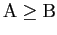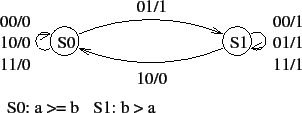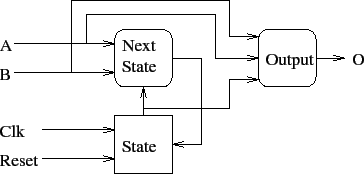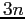# VHDL for Sequential Circuits

Tom Kelliher, CS 240

Apr. 18, 2012

### From Last Time

Sequential circuit design.

### Outline

1. Modified serial comparator.

2. VHDL for serial comparator.

3. Exercise.

Registers

# D Flip Flop

Notes:

1. The flip flop's state is maintained by an internal state signal, qInt.

2. The internal state signal drives the q output.

3. Sequential circuitry should never directly drive an output port.

library IEEE;
use IEEE.STD_LOGIC_1164.ALL;
use IEEE.STD_LOGIC_UNSIGNED.ALL;

entity flipFlop is
Port ( d     : in std_logic;
reset : in std_logic;
clk   : in std_logic;
q     : out std_logic);
end flipFlop;

architecture Behavioral of flipFlop is

signal qInt : std_logic;   -- Maintains FF state.

begin

q <= qInt;   -- Drive FF output from internal state.

-- Trigger state process if clk OR reset changes.

state: process (clk, reset)
begin
-- Asynchronous active low reset.
if reset = '0' then
qInt <= '0';
-- Load new value on rising clock edge.
elsif clk'event and clk = '1' then
qInt <= d;
end if;
end process state;

end Behavioral;


# Modified Serial Comparator

Inputs: A, B, (no more msb). A and B are received least significant bit first. Output 0 if, otherwise 1.

Reset to S0 on reset.

State diagram:# VHDL for Serial Comparator

Things to observe:

1. Flip-flop implementation: reset priority, event, rising edge sensitive.

2. If and case -- sequential statements -- are valid only within a process.

3. Concurrent assignment is a process.''

4. Semantics of a process: sensitivity list, assignments:

b <= a;
c <= b;

does not behave as it would in C.

5. VHDL architecture broken into three processes:
1. State storage.

2. Next state generation.

3. Output generation.Compare process inputs to sensitivity lists.

-- VHDL for serial comparator.  The inputs a and b are input lsb first.
-- The Mealy machine uses rising edge sensitive flip-flops and an
-- asynchronous active low reset.
--
-- The output is 1 if b > a, otherwise 0.

library ieee;
use ieee.std_logic_1164.all;

entity comparator is
port
(a, b, clk, reset : in std_logic;
o                : out std_logic
);
end comparator;

architecture process_defn of comparator is

-- Two states needed.
type state_type is (S0, S1);
-- State assignment.
attribute enum_encoding : string;
attribute enum_encoding of state_type :
type is "0 1";

signal state, next_state : state_type;

-- For convenience, concatenate a and b.
signal inputs : std_logic_vector (1 downto 0);

begin

-- Concurrent assignment executes the rhs changes.
-- Concatenate a and b into inputs.
inputs <= a & b;

-- Processes execute whenever something on their sensitivity list
-- changes.  All assignments take place when the process exits.
--
-- This process implements the D flip-flop.

state_register : process (clk, reset)
begin
-- If/else construct only valid within a process.
if (reset = '0') then
state <= S0;
elsif (clk'event AND clk = '1') then
state <= next_state;
end if;
end process;

-- This process computes the next state.

next_state_process : process (inputs, state)
begin
case state is

when S0 =>
if (inputs = "01") then
next_state <= S1;
else
next_state <= S0;
end if;

when S1 =>
if (inputs = "10") then
next_state <= S0;
else
next_state <= S1;
end if;

end case;
end process;

-- This process computes the output.

output_process : process (inputs, state)
begin
case state is

when S0 =>
if (inputs = "01") then
o <= '1';
else
o <= '0';
end if;

when S1 =>
if (inputs = "10") then
o <= '0';
else
o <= '1';
end if;

end case;
end process;

end process_defn;


# Exercises

1. Serial comparator. Inputs: A, B. A and B are received most significant bit first. Reset to initial state on reset. Output 0 if, otherwise 1.

2. Serialcircuit. Design and use D FF and one bit full adder components.

Thomas P. Kelliher 2012-04-17
Tom Kelliher